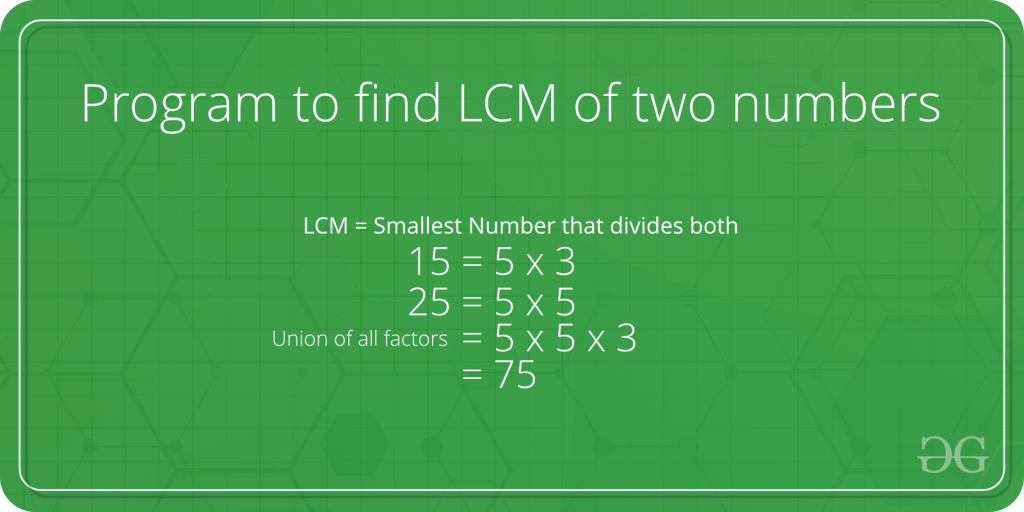Open in App
Not now

# TCS Coding Practice Question | LCM of 2 Numbers

• Difficulty Level : Basic
• Last Updated : 11 Jan, 2023

Given two numbers, the task is to find the LCM of two numbers using Command Line Arguments. LCM (Least Common Multiple) of two numbers is the smallest number which can be divided by both numbers. For example, LCM of 15 and 20 is 60 and LCM of 5 and 7 is 35.Examples:

```Input: n1 = 10, n2 = 15
Output: 30

Input: n1 = 5, n2 = 10
Output: 10```

Approach:

• Since the numbers are entered as Command line Arguments, there is no need for a dedicated input line
• Extract the input numbers from the command line argument
• This extracted numbers will be in string type.
• Convert these numbers into integer type and store it in variables, say num1 and num2
• Find the LCM of the numbers. An efficient solution is based on the below formula for LCM of two numbers ‘a’ and ‘b’.
```   a x b = LCM(a, b) * GCD (a, b)

LCM(a, b) = (a x b) / GCD(a, b) ```
• To find the GCD or HCF, an efficient solution is to use Euclidean algorithm, which is the main algorithm used for this purpose. The idea is, GCD of two numbers doesn’t change if a smaller number is subtracted from a bigger number.
• Print or return the LCM

Program:

## C

 `// C program to compute the LCM of two numbers ` `// using command line arguments ` ` `  `#include ` `#include /* atoi */ ` ` `  `// Function to compute the GCD of two numbers ` `int` `GCD(``int` `a, ``int` `b) ` `{ ` `    ``if` `(b == 0) ` `        ``return` `a; ` ` `  `    ``return` `GCD(b, a % b); ` `} ` ` `  `// Function to find the LCM ` `int` `LCM(``int` `a, ``int` `b) ` `{ ` `    ``return` `(a * b) / GCD(a, b); ` `} ` ` `  `// Driver code ` `int` `main(``int` `argc, ``char``* argv[]) ` `{ ` ` `  `    ``int` `num1, num2; ` ` `  `    ``// Check if the length of args array is 1 ` `    ``if` `(argc == 1) ` `        ``printf``(``"No command line arguments found.\n"``); ` ` `  `    ``else` `{ ` ` `  `        ``// Get the command line argument and ` `        ``// Convert it from string type to integer type ` `        ``// using function "atoi( argument)" ` `        ``num1 = ``atoi``(argv); ` `        ``num2 = ``atoi``(argv); ` ` `  `        ``// Find the LCM and print it ` `        ``printf``(``"%d\n"``, LCM(num1, num2)); ` `    ``} ` `    ``return` `0; ` `} `

## Java

 `// Java program to compute the LCM of two numbers ` `// using command line arguments ` ` `  `class` `GFG { ` ` `  `    ``// Function to compute the GCD of two numbers ` `    ``static` `int` `GCD(``int` `a, ``int` `b) ` `    ``{ ` `        ``if` `(b == ``0``) ` `            ``return` `a; ` ` `  `        ``return` `GCD(b, a % b); ` `    ``} ` ` `  `    ``// Function to find the LCM ` `    ``static` `int` `LCM(``int` `a, ``int` `b) ` `    ``{ ` `        ``return` `(a * b) / GCD(a, b); ` `    ``} ` ` `  `    ``// Driver code ` `    ``public` `static` `void` `main(String[] args) ` `    ``{ ` ` `  `        ``// Check if length of args array is ` `        ``// greater than 0 ` `        ``if` `(args.length > ``0``) { ` ` `  `            ``// Get the command line argument and ` `            ``// Convert it from string type to integer type ` `            ``int` `num1 = Integer.parseInt(args[``0``]); ` `            ``int` `num2 = Integer.parseInt(args[``1``]); ` ` `  `            ``// Find the LCM ` `            ``int` `res = LCM(num1, num2); ` ` `  `            ``// Print the LCM ` `            ``System.out.println(res); ` `        ``} ` `        ``else` `            ``System.out.println(``"No command line "` `                               ``+ ``"arguments found."``); ` `    ``} ` `} `

Output:

Time Complexity : O(logN)

Auxiliary Space: O(logN)

My Personal Notes arrow_drop_up
Related Articles